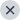008 June, 2022

If you are preparing for the Instrumentation Engineering Paper for the GATE 23, this is the scope of the syllabus that you would need to cover. The order of the topics is random and is not based on the weight of the topic. For any further details, you may get in touch with your nearest center and set up an interaction with a mentor.

Section 1: Engineering Mathematics

Linear Algebra: Matrix algebra, systems of linear equations, consistency and rank, Eigen value and Eigen vectors.

Calculus: Mean value theorems, theorems of integral calculus, partial derivatives, maxima and minima, multiple integrals, Fourier series, vector identities, line, surface and volume integrals, Stokes, Gauss and Green’s theorems.

Differential equations: First order equation (linear and nonlinear), second order linear differential equations with constant coefficients, method of variation of parameters, Cauchy’s and Euler’s equations, initial and boundary value problems, solution of partial differential equations: variable separable method. Analysis of complex variables: Analytic functions, Cauchy’s integral theorem and integral formula, Taylor’s and Laurent’s series, residue theorem, solution of integrals.

Probability and Statistics: Sampling theorems, conditional probability, mean, median, mode, standard deviation and variance; random variables: discrete and continuous distributions: normal, Poisson and binomial distributions.

Numerical Methods: Matrix inversion, solutions of non-linear algebraic equations, iterative methods for solving differential equations, numerical integration, regression and correlation analysis.

Section 2: Electricity and Magnetism

Coulomb’s Law, Electric Field Intensity, Electric Flux Density, Gauss’s Law, Divergence, Electric field and potential due to point, line, plane and spherical charge distributions, Effect of dielectric medium, Capacitance of simple configurations, Biot-Savart’s law, Ampere’s law, Curl, Faraday’s law, Lorentz force, Inductance, Magnetomotive force, Reluctance, Magnetic circuits, Self and Mutual inductance of simple configurations.

Section 3: Electrical Circuits and Machines

Voltage and current sources: independent, dependent, ideal and practical; v-I relationships of resistor, inductor, mutual inductance and capacitor; transient analysis of RLC circuits with dc excitation. Kirchhoff’s laws, mesh and nodal analysis, superposition, Thevenin, Norton, maximum power transfer and reciprocity theorems. Peak-, average- and rms values of ac quantities; apparent-, active- and reactive powers; phasor analysis, impedance and admittance; series and parallel resonance, locus diagrams, realization of basic filters with R, L and C elements. transient analysis of RLC circuits with ac excitation. One-port and two-port networks, driving point impedance and admittance, open-, and short circuit parameters.

Single phase transformer: equivalent circuit, phasor diagram, open circuit and short circuit tests, regulation and efficiency; Three phase induction motors: principle of operation, types, performance, torque-speed characteristics, no-load and blocked rotor tests, equivalent circuit, starting and speed control; Types of losses and efficiency calculations of electric machines.

Section 4: Signals and Systems

Periodic, aperiodic and impulse signals; Laplace, Fourier and z-transforms; transfer function, frequency response of first and second order linear time invariant systems, impulse response of systems; convolution, correlation. Discrete time system: impulse response, frequency response, pulse transfer function; DFT and FFT; basics of IIR and FIR filters.

Section 5: Control Systems

Feedback principles, signal flow graphs, transient response, steady-state-errors, bode plot, phase and gain margins, Routh and Nyquist criteria, root loci, design of lead, lag and lead-lag compensators, state-space representation of systems; time-delay systems; mechanical, hydraulic and pneumatic system components, synchro pair, servo and stepper motors, servo valves; on-off, P, PI, PID, cascade, feedforward, and ratio controllers, tuning of PID controllers and sizing of control valves.

Section 6: Analog Electronics

Characteristics and applications of diode, Zener diode, BJT and MOSFET; small signal analysis of transistor circuits, feedback amplifiers. Characteristics of ideal and practical operational amplifiers; applications of opamps: adder, subtractor, integrator, differentiator, difference amplifier, instrumentation amplifier, precision rectifier, active filters, oscillators, signal generators, voltage-controlled oscillators and phase locked loop, sources and effects of noise and interference in electronic circuits.

Section 7: Digital Electronics

Combinational logic circuits, minimization of Boolean functions. IC families: TTL and CMOS. Arithmetic circuits, comparators, Schmitt trigger, multi-vibrators, sequential circuits, flipflops, shift registers, timers and counters; sample-and-hold circuit, multiplexer, analog-to-digital (successive approximation, integrating, flash and sigma-delta) and digital-to-analog converters (weighted R, R- Copyright © GATE 2022, Indian Institute of Technology Kharagpur 104 of 171 2R ladder and current steering logic). Characteristics of ADC and DAC (resolution, quantization, significant bits, conversion/settling time); basics of number systems, Embedded Systems: Microprocessor and microcontroller applications, memory and input-output interfacing; basics of data acquisition systems, basics of distributed control systems (DCS) and programmable logic controllers (PLC).

Section 8: Measurements

SI units, standards (R, L, C, voltage, current and frequency), systematic and random errors in measurement, expression of uncertainty – accuracy and precision, propagation of errors, linear and weighted regression. Bridges: Wheatstone, Kelvin, Megohm, Maxwell, Anderson, Schering and Wien for measurement of R, L, C and frequency, Q-meter. Measurement of voltage, current and power in single and three phase circuits; ac and dc current probes; true rms meters, voltage and current scaling, instrument transformers, timer/counter, time, phase and frequency measurements, digital voltmeter, digital mustimeter; oscilloscope, shielding and grounding.

Section 9: Sensors and Industrial Instrumentation

Resistive-, capacitive-, inductive-, piezoelectric-, Hall effect sensors and associated signal conditioning circuits; transducers for industrial instrumentation: displacement (linear and angular), velocity, acceleration, force, torque, vibration, shock, pressure (including low pressure), flow (variable head, variable area, electromagnetic, ultrasonic, turbine and open channel flow meters) temperature (thermocouple, bolometer, RTD (3/4 wire), thermistor, pyrometer and semiconductor); liquid level, pH, conductivity and viscosity measurement. 4-20 mA two-wire transmitter.

Section 10: Communication and Optical Instrumentation

Amplitude- and frequency modulation and demodulation; Shannon’s sampling theorem, pulse code modulation; frequency and time division multiplexing, amplitude-, phase-, frequency-, quadrature amplitude, pulse shift keying for digital modulation; optical sources and detectors: LED, laser, photodiode, light dependent resistor, square law detectors and their characteristics; interferometer: applications in metrology; basics of fibre optic sensing. UV-VIS Spectro photometers, Mass spectrometer.